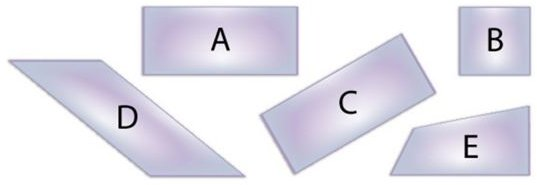# 3rd Grade Math Geometry Lesson PlanComparing and contrasting shapes requires that students use thinking skills even if they don’t realize it!

Objective:

Understand that shapes in different categories (e.g., rhombuses, rectangles, and others) may share attributes (e.g., having four sides), and that the shared attributes can define a larger category (e.g., quadrilaterals). Recognize rhombuses, rectangles, and squares as examples of quadrilaterals, and draw examples of quadrilaterals that do not belong to any of these subcategories. MA3-G-A-1

Materials:

• construction paper
• rulers
• pencils
• scissors

Definitions:

• Quadrilateral: A four sided polygon. (a closed plane figure with edges that are line segments).
• Trapezoid: A quadrilateral with exactly one pair of parallel sides.
• Parallelogram: A four-sided figure with two pairs of parallel sides. Each pair of parallel sides is the same size.
• Rhombus: A parallelogram with four sides the same size (congruent)
• Rectangle: A parallelogram with four right angles
• Square: A rectangle with four congruent sides

## Procedure:

Review the above terms and provide any visual examples that you may have. Using the materials listed above students should follow these directions. Remind them to conserve space on the paper and write each figure’s name in the center of it.:

• Draw a 4” square.
• Draw a rectangle with a pair of 2” sides and a pair of 3”sides.
• Draw a rhombus with 2 ½” sides.
• Draw a trapezoid with the parallel sides being 3 ½”.
• Draw a parallelogram with 1 ½” sides.
• Draw a quadrilateral figure that is not a parallelogram, any size.
• Cut out all figures.

Next instruct students to place the figures in front of them and be ready to listen and hold up the correct figure. Then say things like:

• Hold up a figure that has two pairs of parallel sides (can be a rectangle, square or rhombus) Ask students to explain their choices.
• Hold up a figure with two pairs of parallel sides and all sides are the same length (rhombus or square).
• Hold up a quadrilateral figure that is not a parallelogram (trapezoid or the last figure they drew).
• Hold up two figures that must have four right angles (square and rectangle).
• Hold up a figure that has four equal sides but no right angles. (rhombus).

It’s important to take time for students to explain their choices when answers vary. Remind students of the class rules to remain respectful as they participate in the discussion.

## Extra Activity:

Use the figures that students made to create individual artwork by designing and gluing them on a plain piece of paper. Trading figures is acceptable. Use crayons or markers to add details.